一文了解 Consistent Hash

2019/11/25 14:07

一、数据分布接口定义

概述

1. 有一个分布式环境中唯一的中心分发节点，每次在数据存储的时候，都会询问中心节点这个数据该去哪儿，这个分发节点明确告诉这个数据该去哪儿。

2. 通过一定规则产生一个key，对这个key进行一定规则的运算，得出这个数据该去哪儿。本文描述的取模算法和一致性Hash，就是这样一种方式。

接口定义

/**
* 数据分布hash算法接口定义
* @author xingchuan.qxc
*
*/
public interface HashNodeService {

/**
* 集群增加一个数据存储节点
* @param node
*/

/**
* 数据存储时查找具体使用哪个节点来存储
* @param key
* @return
*/
public Node lookupNode(String key);

/**
* hash的算法
* @param key
* @return
*/
public Long hash(String key);

/**
* 模拟意外情况断掉一个节点，用于测试缓存命中率
* @param node
*/
public void removeNodeUnexpected(Node node);
}

二、数据分布算法实现——取模算法

概述

1. 通过用户的key来取一个hash值

2. 通过这个hash值来对存储节点数n进行取模，得出一个index

3. 上面这个index就是待存储的节点标识

Note：本文例子我生成hash值的方式，我采用CRC32的方式。

代码实现：

/**
* 取模数据分布算法实现
* @author xingchuan.qxc
*
*/
public class NormalHashNodeServiceImpl implements HashNodeService{

/**
* 存储节点列表
*/
private List<Node> nodes = new ArrayList<>();

@Override
}
@Override
public Node lookupNode(String key) {
long k = hash(key);
int index = (int) (k % nodes.size());
return nodes.get(index);
}
@Override
public Long hash(String key) {
CRC32 crc32 = new CRC32();
crc32.update(key.getBytes());
return crc32.getValue();
}
@Override
public void removeNodeUnexpected(Node node) {
nodes.remove(node);
}
}

HashNodeService nodeService = new NormalHashNodeServiceImpl();
Node addNode1 = new Node("xingchuan.node1", "192.168.0.11");
Node addNode2 = new Node("xingchuan.node2", "192.168.0.12");
Node addNode3 = new Node("xingchuan.node3", "192.168.0.13");
Node addNode4 = new Node("xingchuan.node4", "192.168.0.14");
Node addNode5 = new Node("xingchuan.node5", "192.168.0.15");
Node addNode6 = new Node("xingchuan.node6", "192.168.0.16");
Node addNode7 = new Node("xingchuan.node7", "192.168.0.17");
Node addNode8 = new Node("xingchuan.node8", "192.168.0.18");

//用于检查数据分布情况
Map<String, Integer> countmap = new HashMap<>();
Node node = null;
for (int i = 1; i <= 100000; i++) {
String key = String.valueOf(i);
node = nodeService.lookupNode(key);
node.cacheString(key, "TEST_VALUE");
String k = node.getIp();
Integer count = countmap.get(k);
if (count == null) {
count = 1;
countmap.put(k, count);
} else {
count++;
countmap.put(k, count);
}

}
System.out.println("初始化数据分布情况：" + countmap);

{
192.168.0.11=12499,
192.168.0.12=12498,
192.168.0.13=12500,
192.168.0.14=12503,
192.168.0.15=12500,
192.168.0.16=12502,
192.168.0.17=12499,
192.168.0.18=12499
}

缺点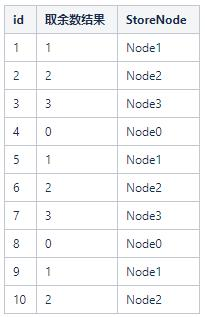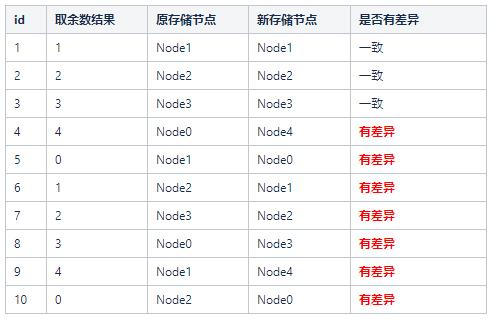HashNodeService nodeService = new NormalHashNodeServiceImpl();
Node addNode1 = new Node("xingchuan.node1", "192.168.0.11");
Node addNode2 = new Node("xingchuan.node2", "192.168.0.12");
Node addNode3 = new Node("xingchuan.node3", "192.168.0.13");
Node addNode4 = new Node("xingchuan.node4", "192.168.0.14");
Node addNode5 = new Node("xingchuan.node5", "192.168.0.15");
Node addNode6 = new Node("xingchuan.node6", "192.168.0.16");
Node addNode7 = new Node("xingchuan.node7", "192.168.0.17");
Node addNode8 = new Node("xingchuan.node8", "192.168.0.18");

//用于检查数据分布情况
Map<String, Integer> countmap = new HashMap<>();
Node node = null;
for (int i = 1; i <= 100000; i++) {
String key = String.valueOf(i);
node = nodeService.lookupNode(key);
node.cacheString(key, "TEST_VALUE");
String k = node.getIp();
Integer count = countmap.get(k);
if (count == null) {
count = 1;
countmap.put(k, count);
} else {
count++;
countmap.put(k, count);
}

}
System.out.println("初始化数据分布情况：" + countmap);
// 正常情况下的去获取数据，命中率
int hitcount = 0;
for (int i = 1; i <= 100000; i++) {
String key = String.valueOf(i);
node = nodeService.lookupNode(key);
if (node != null) {
String value = node.getCacheValue(key);
if (value != null) {
hitcount++;
}
}
}
double h = Double.parseDouble(String.valueOf(hitcount))/ Double.parseDouble(String.valueOf(100000));
System.out.println("初始化缓存命中率:"+ h);
// 移除一个节点
Node addNode9 = new Node("xingchuan.node0", "192.168.0.19");
hitcount = 0;
for (int i = 1; i <= 100000; i++) {
String key = String.valueOf(i);
node = nodeService.lookupNode(key);
if (node != null) {
String value = node.getCacheValue(key);
if (value != null) {
hitcount++;
}
}
}
h = Double.parseDouble(String.valueOf(hitcount))
/ Double.parseDouble(String.valueOf(100000));
System.out.println("增加一个节点后缓存命中率:"+ h);

{
192.168.0.11=12499,
192.168.0.12=12498,
192.168.0.13=12500,
192.168.0.14=12503,
192.168.0.15=12500,
192.168.0.16=12502,
192.168.0.17=12499,
192.168.0.18=12499
}

三、分布式数据分布算法——一致性Hash

概述

1. 想象有一个巨大的环，比如这个环的值的分布可以是 0 ~ 4294967296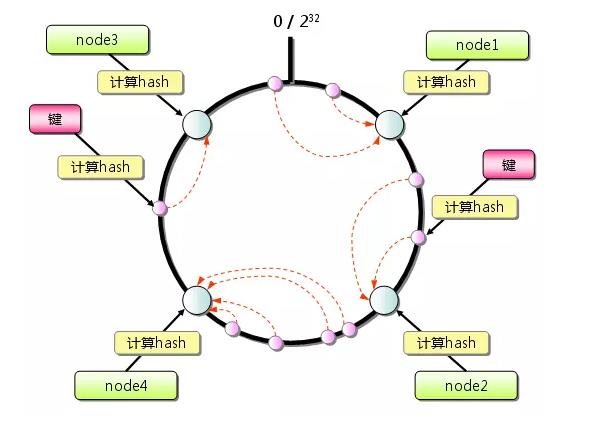2. 还是在取模算法中的那个例子，这时候我们假定我们的4个节点通过一些key的hash，分布在了这个巨大的环上面。

3. 用户数据来了，需要存储到哪个节点呢？通过key的hash，得出一个值r，顺时针找到最近的一个Node节点对应的hash值nodeHash，这次用户数据也就存储在对应的这个Node上。

一致性Hash的实现

一致性Hash实现，进阶一，关键代码如下：

@Override
long crcKey = hash(node.getIp());
nodeMap.put(crcKey, node);
}

@Override
public Node lookupNode(String key) {
long crcKey = hash(key);
Node node = findValidNode(crcKey);
if(node == null){
return findValidNode(0);
}
return node;
}

/**
* @param crcKey
*/
private Node findValidNode(long crcKey) {
//顺时针找到最近的一个节点
Map.Entry<Long,Node> entry = nodeMap.ceilingEntry(crcKey);
if(entry != null){
return entry.getValue();
}
return null;
}

@Override
public Long hash(String key) {
CRC32 crc = new CRC32();
crc.update(key.getBytes());
return crc.getValue();
}

测试代码如下：

HashNodeService nodeService = new ConsistentHashNodeServiceImpl();

{
192.168.0.11=2495,
192.168.0.12=16732,
192.168.0.13=1849,
192.168.0.14=32116,
192.168.0.15=2729,
192.168.0.16=1965,
192.168.0.17=38413,
192.168.0.18=3701
}

一致性Hash的实现，进阶2，引入虚拟节点，代码如图：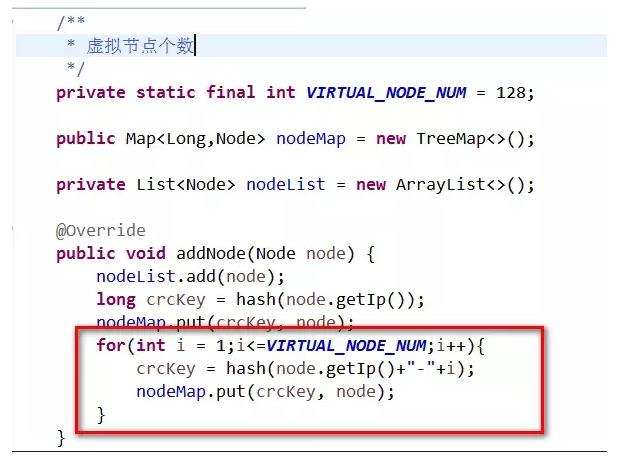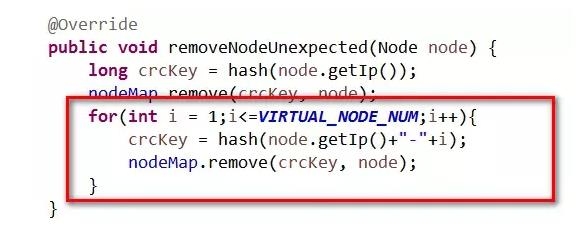再次测试数据分布和缓存命中率

{
192.168.0.11=11610,
192.168.0.12=14600,
192.168.0.13=13472,
192.168.0.14=11345,
192.168.0.15=11166,
192.168.0.16=12462,
192.168.0.17=14477,
192.168.0.18=10868
}

总结0
6 收藏

作者的其它热门文章0 评论
6 收藏
0